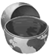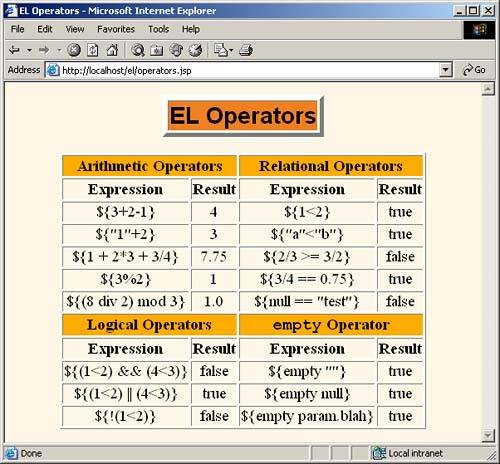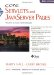# 16.9 Using Expression Language Operators

The JSP 2.0 expression language defines a number of arithmetic, relational, logical, and missing-value-testing operators. Although use of the operators often results in code that is a bit shorter than the equivalent Java code, you should keep in mind the main purpose of the expression language: to provide concise access to existing objects, usually in the context of the MVC architecture. So, we see little benefit in replacing long and complex scripting elements with only slightly shorter expression language elements. Both approaches are wrong; complex business- or data-access -logic does not belong in JSP pages at all. As much as possible, restrict the use of the expression language to presentation logic; keep the business logic in normal Java classes that are invoked by servlets.

Core ApproachUse the expression language operators for simple tasks oriented toward presentation logic (deciding how to present the data). Avoid using the operators for business logic (creating and processing the data). Instead, put business logic in regular Java classes and invoke the code from the servlet that starts the MVC process.

#### Arithmetic Operators

These operators are typically used to perform simple manipulation of values already stored in beans. Resist using them for complex algorithms; put such code in regular Java code instead.

+ and

These are the normal addition and subtraction operators, with two exceptions. First, if either of the operands is a string, the string is automatically parsed to a number (however, the system does not automatically catch NumberFormatException ). Second, if either of the operands is of type BigInteger or BigDecimal , the system uses the corresponding add and subtract methods .

* , /, and div

These are the normal multiplication and division operators, with a few exceptions. First, types are converted automatically as with the + and operators. Second, the normal arithmetic operator precedence applies, so, for instance,

` `
` \${ 1 + 2 * 3} `

returns 7, not 9. You can use parentheses to change the operator precedence. Third, the / and div operators are equivalent; both are provided for the sake of compatibility with both XPath and JavaScript (ECMAScript).

% and mod

The % (or equivalently, mod ) operator computes the modulus (remainder), just as with % in the Java programming language.

#### Relational Operators

These operators are most frequently used with either the JSP expression language conditional operator (Section 16.10) or with custom tags whose attributes expect boolean values (e.g., looping tags like those in the JSP Standard Tag LibraryJSTLas discussed in Volume 2 of this book).

== and eq

These two equivalent operators check whether the arguments are equal. However, they operate more like the Java equals method than the Java == operator. If the two operands are the same object, true is returned. If the two operands are numbers , they are compared with Java == . If either operand is null , false is returned. If either operand is a BigInteger or BigDecimal , the operands are compared with compareTo . Otherwise, the operands are compared with equals .

!= and ne

These two equivalent operators check whether the arguments are different. Again, however, they operate more like the negation of the Java equals method than the Java != operator. If the two operands are the same object, false is returned. If the two operands are numbers, they are compared with Java != . If either operand is null , true is returned. If either operand is a BigInteger or BigDecimal , the operands are compared with compareTo . Otherwise, the operands are compared with equals and the opposite result is returned.

< and lt, > and gt, <= and le, >= and ge

These are the standard arithmetic operators with two exceptions. First, type conversions are performed as with == and != . Second, if the arguments are strings, they are compared lexically.

#### Logical Operators

These operators are used to combine results from the relational operators.

&&, and, , or, !, not

These are the standard logical AND, OR, and NOT operators. They operate by coercing their arguments to Boolean , and they use the normal Java "short circuit" evaluation in which the testing is stopped as soon as the result can be determined. && and and are equivalent, and or are equivalent, and ! and not are equivalent.

#### The empty Operator

empty

This operator returns true if its argument is null , an empty string, an empty array, an empty Map , or an empty collection. Otherwise it returns false .

#### An Example

Listing 16.11 illustrates several of the standard operators; Figure 16-6 shows the result.

##### Listing 16.11 operators.jsp
` <!DOCTYPE HTML PUBLIC "-//W3C//DTD HTML 4.0 Transitional//EN"> <HTML> <HEAD><TITLE>EL Operators</TITLE> <LINK REL=STYLESHEET       HREF="/el/JSP-Styles.css"       TYPE="text/css"> </HEAD> <BODY> <TABLE BORDER=5 ALIGN="CENTER">   <TR><TH CLASS="TITLE">   EL Operators </TABLE> <P> <TABLE BORDER=1 ALIGN="CENTER">   <TR><TH CLASS="COLORED" COLSPAN=2>Arithmetic Operators       <TH CLASS="COLORED" COLSPAN=2>Relational Operators   <TR><TH>Expression<TH>Result<TH>Expression<TH>Result   <TR ALIGN="CENTER">     <TD>\${3+2-1}<TD>\${3+2-1}  <%-- Addition/Subtraction --%>     <TD>\${1&lt;2}<TD>\${1<2}      <%-- Numerical comparison --%>   <TR ALIGN="CENTER">     <TD>\${"1"+2}<TD>\${"1"+2}     <%-- String conversion --%>     <TD>\${"a"&lt;"b"}<TD>\${"a"<"b"} <%-- Lexical comparison --%>   <TR ALIGN="CENTER">     <TD>\${1 + 2*3 + 3/4}<TD>\${1 + 2*3 + 3/4}  <%-- Mult/Div --%>     <TD>\${2/3 &gt;= 3/2}<TD>\${2/3 >= 3/2}        <%-- >= --%>   <TR ALIGN="CENTER">     <TD>\${3%2}<TD>\${3%2}                 <%-- Modulo --%>     <TD>\${3/4 == 0.75}<TD>\${3/4 == 0.75} <%-- Numeric = --%>   <TR ALIGN="CENTER">     <%-- div and mod are alternatives to / and % --%>     <TD>\${(8 div 2) mod 3}<TD>\${(8 div 2) mod 3}     <%-- Compares with "equals" but returns false for null --%>     <TD>\${null == "test"}<TD>\${null == "test"}   <TR><TH CLASS="COLORED" COLSPAN=2>Logical Operators       <TH CLASS="COLORED" COLSPAN=2><CODE>empty</CODE> Operator   <TR><TH>Expression<TH>Result<TH>Expression<TH>Result   <TR ALIGN="CENTER">     <TD>\${(1&lt;2) && (4&lt;3)}<TD>\${(1<2) && (4<3)} <%--AND--%>     <TD>\${empty ""}<TD>\${empty ""} <%-- Empty string --%>   <TR ALIGN="CENTER">     <TD>\${(1&lt;2)  (4&lt;3)}<TD>\${(1<2)  (4<3)} <%--OR--%>     <TD>\${empty null}<TD>\${empty null} <%-- null --%>   <TR ALIGN="CENTER">     <TD>\${!(1&lt;2)}<TD>\${!(1<2)}  <%-- NOT -%>     <%-- Handles null or empty string in request param --%>     <TD>\${empty param.blah}<TD>\${empty param.blah} </TABLE> </BODY></HTML> `
##### Figure 16-6. The expression language defines a small set of operators. Use them with great restraint; invoke business logic from servlets, not from JSP pages.Core Servlets and Javaserver Pages: Core Technologies, Vol. 1 (2nd Edition)
ISBN: 0130092290
EAN: 2147483647
Year: 2002
Pages: 194

Similar book on Amazon